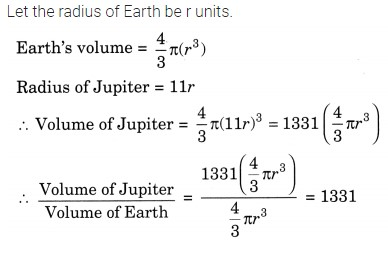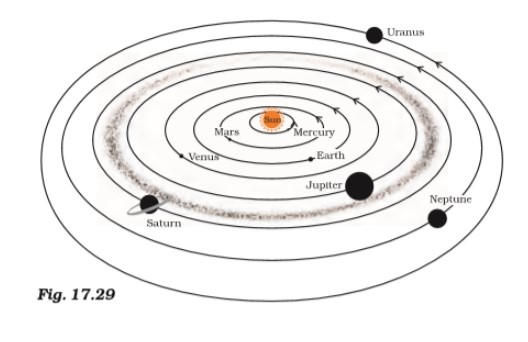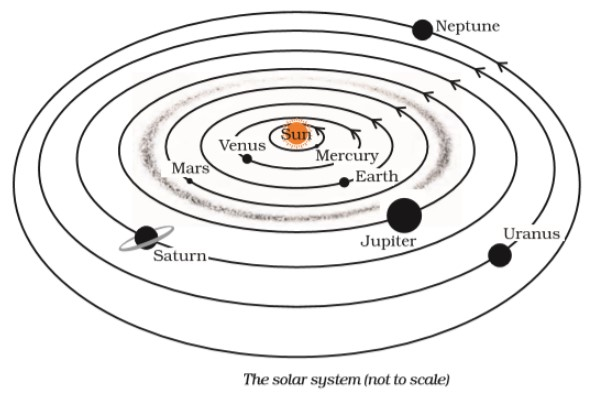# NCERT Solutions for Class 8 Science Chapter 17 Stars and the Solar System

Exercises

NCERT Solutions for Class 8 Science Chapter 17 Stars and the Solar System

Choose the correct answer in Questions 1-3.

Question:- 1. Which of the following is NOT a member of the solar system?

(a) An asteroid

(b) A satellite

(c) A constellation

(d) A comet

Answer:- (c) A constellation

Question:- 2. Which of the following is NOT a planet of the sun?

(a) Sirius

(b) Mercury

(c) Saturn

(d) Earth

Question:- 3. Phases of the moon occur because

(a) we can see only that part of the moon which reflects light towards us.

(b) our distance from the moon keeps changing.

(c) the shadow of the Earth covers only a part of the moon’s surface.

(d) the thickness of the moon’s atmosphere is not constant.

Answer:- (a) we can see only that part of the moon which reflects light towards us.

Question:- 4. Fill in the blanks.

(a) The planet which is farthest from the Sun is ____________ .

(b) The planet which appears reddish in colour is ____________ .

(c) A group of stars that appear to form a pattern in the sky is known as a ____________ .

(d) A celestial body that revolves around a planet is known as __________.

(e) Shooting stars are actually not ____________.

(f) Asteroids are found between the orbits of _________ and _________ .

Answer:- (a) The planet which is farthest from the Sun is Neptune.

(b) The planet which appears reddish in colour is Mars .

(c) A group of stars that appear to form a pattern in the sky is known as a Constellation .

(d) A celestial body that revolves around a planet is known as satellite

(e) Shooting stars are actually not stars.

(f) Asteroids are found between the orbits of Mars and Jupiter .

Question:- 5. Mark the following statements as true (T) or false (F).

(a) Pole star is a member of the solar system. ( )

(b) Mercury is the smallest planet of the solar system. ( )

(c) Uranus is the farthest planet in the solar system. ( )

(d) INSAT is an artificial satellite. ( )

(e) There are nine planets in the solar system. ( )

(f) Constellation Orion can be seen only with a telescope. ( )

(a) False

(b) True

(c) False

(d) True

(e) False

(f) False

Question:- 6. Match items in column A with one or more items in column B.

 A B (i) Inner planets (a) Saturn (ii) Outer planets (b) Pole star (iii) Constellation (c) Great Bear (iv) Satellite of the Earth (d) Moon (e) Earth (f) Orion (g) Mars

 A B (i) Inner planets (g) Mars (e) Earth (ii) Outer planets (a) Saturn (iii) Constellation (c) Great Bear (f) Orion (iv) Satellite of the Earth (d) Moon

Question:- 7. In which part of the sky can you find Venus if it is visible as an evening star?

Answer:-  At west side of the sky we can find Venus if it is visible as an evening star.

Question:- 8. Name the largest planet of the solar system.

Answer:- Jupiter is the largest planet of the solar system.

Question:- 9. What is a constellation? Name any two constellations.

Answer:- Constellations are groups of stars that appear to form well-known or recognisable shapes. Great Bear and Orion are two example of constellations.

Question:- 10. Draw sketches to show the relative positions of prominent stars in

(a) Ursa Major     and           (b) Orion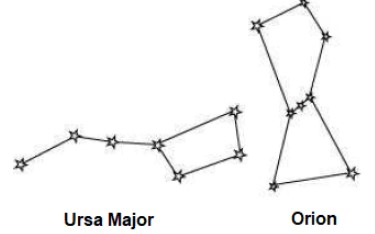Question:- 11. Name two objects other than planets which are members of the solar system.

Answer:-   Meteors and asteroids are two objects other than planets which are members of the solar system.

Question:- 12. Explain how you can locate the Pole Star with the help of Ursa Major.

Answer:- Two stars near the end of Ursa Major can be used to find the Pole Star. Consider drawing a straight line through these stars. Extend the imaginary line in the direction of the north pole. This line spans the distance between two stars by nearly five times. In this direction, a star known as the Pole star can be observed.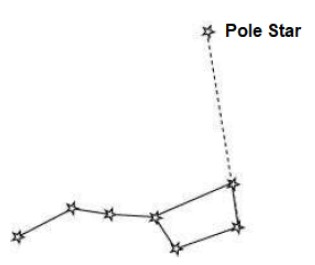Question:- 13. Do all the stars in the sky move? Explain.

Answer:-  No, the stars in the sky do not all move. Stars appear to move in the sky due to the Earth’s revolution on its own axis.

Question:- 14. Why is the distance between stars expressed in light years? What do you understand by the statement that a star is eight light years away from the Earth?

Answer:-  The stars are quite far away from the Earth, and expressing such distances in kilometres is difficult. As a result, such vast distances are measured in light years. When we say that a star is eight light years away from Earth, we indicate that the star’s light will take eight years to reach us.

Question:- 15. The radius of Jupiter is 11 times the radius of the Earth. Calculate the ratio of the volumes of Jupiter and the Earth. How many Earths can Jupiter accomodate?